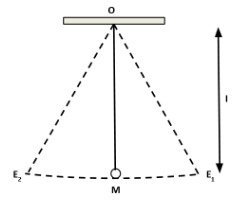# How is the time period $T$ and frequency $f$ of a simple pendulum related to each other?Verified
143.7k+ views
Hint: Simple pendulums have oscillatory and periodic motions. Time period is defined as the time taken by the bob of a pendulum to finish a left swing and a right swing of. Frequency is defined as the number of oscillations made by the bob of the pendulum per second. The frequency and time period of an oscillation are inversely related to each other.The distance between the point of suspension $O$ and the center of gravity $g$ of the bob is called the length of the simple pendulum. It is denoted by $l$ . Then the time period of the pendulum is given by
$T = 2\pi \sqrt {\dfrac{l}{g}}$
Therefore, $f = \dfrac{1}{T} = \dfrac{1}{{2\pi }}\sqrt {\dfrac{g}{l}}$
Hence, the time period $T$ and frequency $f$ of a simple pendulum related to each other as $f = \dfrac{1}{T}$.